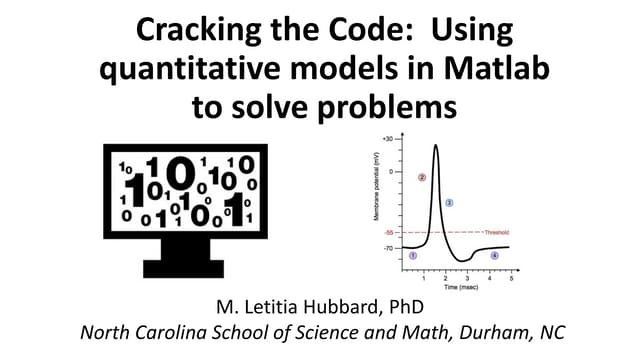# Modeling

Content on this page is derived from participant presentations, discussions, and breakout groups at the Teaching Computation with MATLAB workshops.

Modeling in courses that incorporate computation can help students better understand physical systems. Conceptualizing a model gives students the opportunity to define inputs/outputs, conservative quantities, discretization, and boundary and initial conditions. In addition, students evaluate assumptions and make predictions––important skills transferable through STEM. Workshop participants identified a number of strategies and approaches to help develop students' modeling skills. These strategies include providing students with a strong conceptual foundation, scaffolding activities to ease students in and give feedback, and developing clear learning objectives.

Jump down to: Challenges and Solutions | Resources

## Approaches to Teaching Modeling

During the 2016 workshop, participants identified the following approaches to introduce modeling to students:

• Ask conceptual questions early on and use real life examples
• Explore system behavior before the mathematical underpinnings
• MATLAB SimBiology is one example of a visual environment that instructors can use to get students started with modeling.
• Use pedagogical approaches and best-practices

### Examples from workshop participants:Click to view
Greg Hancock (William & Mary) presented his approach to introducing students to modeling at the 2016 workshop: Introducing Mass Balancing Modeling Using a Controversial Environmental Problem. Hancock focuses on developing clear learning objectives and has the students answer the essential questions to constructing a mass conservation model. Using an environmental issue, water level in Mono Lake, CA, shows students that modeling and MATLAB can help solve real-world problems.

Check out the corresponding teaching activity: Modeling Mono Lake's Water Balance by Phil Resor and Greg Hancock

Greg Hancock's Learning Objectives for Modeling

1. Translation from idea to model construction
2. Identifying and evaluating model assumptions
3. Planning and troubleshooting numerical models
4. Calibration and verification of a model
5. Using models for prediction
6. Presentation of modeling resultClick to view
Letitia Hubbard (North Carolina School of Science and Math) used the Hodgkin-Huxley model as an example of how to help students develop a quantitative model to solve problems. In addition to the presentation, her teaching activity, modeling a neuron action potential in MATLAB, describes the learning goals, assessment, teaching tips, and necessary background information. To train the students to use models, Hubbard has students discern the physical basis for the model and demonstrate they understand the numerical methods by computing them by hand. Then, the students translate equations into MATLAB code to create the computational tool. Using a simulation, they can explore the impact of different variables on the model.

## Common Challenges and Solutions

Working groups considered common challenges to teaching modeling in undergraduate science courses. Some commonly cited challenges include:

Biology

Engineering

Geoscience

Mathematics

Physics